﻿ The Improving Mathematical Communication Ability and Students’ Self-Regulation Learning through Realistic Mathematical Approach Based on Batak Toba CulturePublications are Open
Access in this journal
Article Versions
Export Article
• Normal Style
• MLA Style
• APA Style
• Chicago Style
Research Article
Open Access Peer-reviewed

### The Improving Mathematical Communication Ability and Students’ Self-Regulation Learning through Realistic Mathematical Approach Based on Batak Toba Culture

Aman Sanusi Siregar , Edy Surya, Edi Syahputra, Asril Rais Sirait
American Journal of Educational Research. 2018, 6(10), 1397-1402. DOI: 10.12691/education-6-10-9
Received August 05, 2018; Revised October 14, 2018; Accepted October 28, 2018

### Abstract

This purpose of this research to know: (1) The improving of mathematical communication ability and students’ self regulated learning through realistic mathematics approach based on Batak Toba culture is higher than students who are given regular learning, (2) there is interaction between learning approach with initial ability of mathematic (KAM) in improvement of students mathematical communication ability and students’ self regulated learning. This research type is quasi experiment. This research was conducted at SMP N 2 Dolok Panribuan. The population in this study is all students of class VII. Based on the results of the analysis, the research results are obtained: (1) improving of mathematical communication ability and students’ self regulated learning through realistic mathematics approach based on Batak Toba culture is higher than students who are given regular learning, (2) there is no interaction between learning approach with initial ability of mathematic (KAM) in improvement of students mathematical communication ability and students’ self regulated learning. Recommended by the teachers can use realistic mathematics approach based Batak Toba culture by presenting issues related to local culture as an alternative learning.

### 1. Introduction

Improving the quality of mathematics learning, especially on intensity and quality in learning, will build and develop students' basic mathematical abilities. According Nesusin N. et, all (2014) that the learning process must provide many benefits for students so that they can develop their full potential 1. Through the process of learning mathematics can also foster students' hard skills and mathematical soft skills. Hendriana, H., Rohaeti, E, U., And Sumarmo, U (2017) stated that mathematical communication is one of the hard skills that students must possess because it is an essential mathematical ability that is essential and needs to be possessed by junior high school students. 2 Another thing that underlies that mathematical communication is the essence of teaching, learning, and accessing mathematics (Pressini and Bassett in Izzati and Suryadi, 2010, Lindquist in Taufiq, 2014). 3, 4.

Communication skills are listed in the curriculum and learning objectives of mathematics (NCTM, 2000,). 5 Mathematical communication is the basis for solving problems, so students are able to explore, investigate and formulate into mathematical models. This will build a mathematical mindset so as to be able to express ideas correctly, and convey them into the language of mathematics.

Based on the survey results, students' mathematical communication is still low and unsatisfactory, this is also found (Izzati, N, 2012, Laurens, T., et, all, 2018, Oktavianingstya, E, 2011, Tiffany, F., et, all, 2017, Yusra, DA & Saragih, S, 2016). 6, 7, 8, 9, 10. Indications that cause it are students have difficulty answering questions in the form of verbal, students are not able to express mathematical ideas in writing, difficult to understand the problem and form into mathematical models, this is also found (Izzati, N, 2012, Laurens, T., et, all, 2018, Oktavianingstya, E, 2011, Tiffany, F., et, all, 2017, Yusra, DA & Saragih, S, 2016). 6, 7, 8, 9, 10.

This is the reason for improving students' mathematical communication skills. In order to achieve the maximum mathematical communication skills of students, it must be in line with the soft skills of students. One important factor in supporting this is learning independence. Self Regulated Learning (SRL) is an active and determined process in which students determine learning goals and to monitor, regulate, control their cognition, motivation, and attitude, guided and constrained by their goals, and contextual environmental features (Abubakar, BA, and Mohammad YA, 2015, Pintrich, 1990, Rusman, 2014). 11, 12, 13 Learning progress will be formed from an independent learning process. Independent learning is one indicator that determines the success of student learning (Sumarmo, U. & Sugandi, AS, 2010) 14.

The success of students in learning mathematics is also influenced by readiness and the ability of students to take lessons is also determined by the initial ability of mathematics (KAM) owned by students. Astuti, SP (2015) states that the initial ability of students is the ability that has been owned by students before participating in the learning that will be given. 15. The initial abilities of Mathematics students (KAM) are classified into high, medium and low groups. Early Mathematical ability is a prerequisite that must be possessed by students in order to be able to follow the lesson well and smoothly.

Student learning outcomes cannot be separated from and how teachers teach and students' responses to mathematics it self. During this time the learning is still using the usual approach where the learning process of mathematics is generally done in school (often called traditional learning). Research conducted by Schwerdt and Wuppermann (2010) shows that traditional lecture teaching style shows very high student achievement. Traditional teaching has been going on for thousands of years, shaping the minds of many ordinary scientists, workers, men and women. Traditional learning has become a proven method, but also has several weaknesses. 16.

Although traditional learning is dominantly used in learning, the world of education currently prioritizes student activity in learning (Laurens, T., et, all, 2018, Yusra, DA & Saragih, S, 2016). 7, 10 Mathematical learning today must link the mathematical concepts in school with their daily experiences. Mathematical learning is too formal, has no connection with meaning, understanding, and application of mathematical concepts, and fails to give sufficient attention to reasoning and problem solving abilities (NCTM, 2014). 17.

Mathematical learning is basically designed to provide continuous enjoyment and comfort for students without imposing anything on them (Laurens, T., et, all, 2018) 7. But with a teacher-centered approach, students only see mathematics as a set of rules and exercises that bring boredom. There is no benefit to learning mathematics in life because the activities of students only repeat the procedure or memorize without being given the opportunity to interact more with others, this can give the impression that mathematics is memorized to not learn to work alone Adek, S., et all. 2017. 18 Teaching involves sequential and systematic presentation of facts, ideas, experiences, knowledge, skills, and information by teachers to students. This process is not as simple as is often felt (Amadil, Glory, & Kufre Akpan, 2017) 19. Methode learning mathematics must focus on exploration and mathematical inquiry (Abubakar, BA, 2015) 1.

One of the approaches supporting is Realistic Mathematics Education (PMR). The student learning process will only happens if the knowledge learned by students will be meaningful to the students themselves (Wijaya, A. 2012). 20 A realistic mathematical approach is a learning approach that departs from 'real' things for students, emphasizes skills, discusses and collaborates, debates with classmates so they can find themselves and ultimately uses mathematics to solve problems both individually and in groups.

Compatibility between curriculum and PMR in terms of the purpose of learning mathematics in schools needs to be integrated with the cultural context. Education is part of culture, education and culture have a reciprocal influence. If culture changes maybe education will also change. Cultural context can be used as a tool for learning to motivate students to apply mathematical concepts, work in groups, and perceived relationships between various subjects (Saragih, S., E. Napitupulu, EE & Fauzi, A. 2016). 21 Thus it is possible to have a local cultural context, namely theculture Toba Batak in learning mathematics, meaningful learning processes will occur as expected.

So that education and culture are one of the most important and unavoidable units in everyday life. One culture related to education is the Toba Batak culture. Realistic mathematics learning based on Toba Batak culture will provide content that bridges between formal and informal mathematics, namely mathematics in the everyday world close to the local culture of students. This will give birth to a generation that is competent and dignified, reflects cultural values, participates in shaping the character of the nation, contributes to the creation of national identity, and contributes to preserving the nation's culture. Based on this reason, research is needed to do with the title of an increase in students' mathematical communication skills and independent learning through realistic mathematical approach based Batak Toba culture.

### 2. Methods

This type of research is quasi-experimental research. This research was conducted in SMP N 2 Dolok Panribuan the second semester of the 2017 / 2018 academic year. In this study sample selection conducted using cluster random sampling technique. Cluster random sampling, in this sampling, the population is divided into several groups or clusters. Randomly needed clusters were taken by randomization process. Each member in the randomly drawn clusters is the required sample 19.

Based on random sampling from 9 grade VII students at Dolok Panribuan 2 SMP, students were chosen as samples of 2 classes, namely class VII-2 as an experimental class which was given learning with a realistic approach based on Batak Toba culture and class VII-3 as a control class who are given learning by reguler approach. Each number of students in the two classes is 32 students. As for the research design used in this study is the pretest-posttest control group design. The design of analyze was 2x2 factorial designs using technical analyze of variance (ANOVA) two ways.

The student indicators of mathematical communication skills test consists of 3 aspects: drawing, writing and explaining. Indicators of self-learning are organized into three aspects, namely: Planning, business control in academic classes and cognitive strategies for understanding the material. Data collection techniques were obtained through students' mathematical communication tests and the SRL questionnaire. The instrument has been validated and meets the requirements for testing validity and reliability. Data collection will be carried out in two stages, collecting data about students 'independent learning and collecting data on students' mathematical communication. The design of research were given on Table 1.

Based on the experimental research design, the hypothesis of the experimental equation (experiment) was adapted from Syahputra (2016) as follows: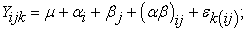22

The research hypothesis is as follows:

a. Hypothesis 1: To examine differences improvement of students' mathematical communication skills.

b. Hypothesis 2: To examine differences improvement of students' self regulated learning.

c. Hypothesis 3: To examine the interaction between learning and KAM to improve students' mathematical communication skills.

d. Hipotesis 4: To examine the interaction between learning and KAM to improve students' self regulated learning.

### 3. Results

Pretest is done to determine students' abilities before applying realistic mathematical approaches based on Batak Toba culture and the reguler approach. Based on the pretest with 10 items obtained in the class realistic mathematics approach based on Batak Toba culture as many as 32 students, there were 7 students who had high ability, 20 students had moderate ability, and 5 students had low ability. Likewise, in the regular approach class there were 32 students, there were 4 students who had high abilities, 22 students had moderate abilities, and 6 students had low abilities. Before the data were analyzed, the normality of the data was tested using chi-square test and homogeneity test used Fisher's homogeneity assumption (Test-F) as a condition of qualitative analysis. The results obtained that the two classes come from populations that are normally distributed and homogen.

Data on students' mathematical communication ability in a realistic mathematics approach class based on Batak Toba culture and regular classes are shown in the following table.

Based on Table 2 it can be seen that in the control class the average pretest score is 54,44 and the average posttest score is 59,37, there is an average difference of 5,52. Whereas in the experimental class the average pretest score is 53,85 and the average posttest value is 67,78, there is an average difference of 13,93. The average N-Gain in the experimental class and the control class have different mean values, ie 0.30 and 0.10, respectively, so it can be seen for the average value of the higher experimental class N-Gain. Descriptively it can be seen that the difference between the average pretest score and the average posttest value in the experimental class is higher than the difference in the average pretest score with the average control class posttest score.

Data on students' self regulated learning in a realistic mathematics approach class based on Batak Toba culture and regular classes are shown in the following table.

Based on Table 3 it can be seen that in the control class the average pretest score is 64, 15 and the average posttest score is 71, 98, there is an average difference of 7, 83. Whereas in the experimental class the average pretest score is 63, 67 and the average posttest value is 81, 90, there is an average difference of 18, 23. The average N-Gain in the experimental class and the control class have different mean values, ie 0.48 and 0.19, respectively, so it can be seen for the average value of the higher experimental class N-Gain. Descriptively it can be seen that the difference between the average pretest score and the average posttest value in the experimental class is higher than the difference in the average pretest score with the average control class posttest score.

After that, it was analyzed using analysis of variance (ANOVA) to test 4 hypotheses proposed in the study with a two-way variance analysis test. The results of data analysis can be seen in the following table:

From the results of the above analysis, two variants can explain some of the following:

1. Based on Table 4 above shows that the value of Fcount between rows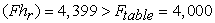which means H0 is rejected. So there is an increase in students' mathematical communication skills that are given learning through a realistic mathematical approach based on Batak Toba culture rather than students who are given learning through the usual approach.

2. Then from Table 4 above also shows that the value of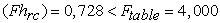thus H0 is accepted. So it can be concluded that there is no interaction between the learning approach and the initial ability of mathematics to students' mathematical communication. This result can also be described through the following Figure 1.

From the results of the above analysis, two variants can explain some of the following:

1. Based on Table 5 above shows that the value of Fcount between rows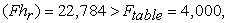which means H0 is rejected. So that there is an increase in learning independence of students who are given learning through a realistic mathematical approach based on Batak Toba culture rather than students who are given learning through the usual approach.

2. Then from Table 5 above also shows that the value of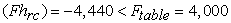thus H0 is accepted. So it can be concluded that there is no interaction between the learning approach and the initial ability of mathematics towards student self regulated learning This result can also be described through the following Figure 2.

• Figure 1. Interaction between learning and KAM on student mathematical communication improvemen
• Figure 2. Interaction between learning and KAM on improve student self regulated learning

### 4. Discussion

4.1. Mathematical Communication Ability

Based on average values, N-Gain and hypothesis testing show that the mathematical communication skills of students with realistic mathematical approaches based on Batak Toba culture are better than using reguler approaches. The results of this study are similarities found in previous studies. Makonye, Yehuda. P. (2014) shows that by applying Realistic Mathematic Educational Approach (RME) when learning helps students to see the close relationship between conceptual knowledge of mathematics and mathematical procedural knowledge. 23 Realistic mathematical approaches based on Toba Batak culture provide opportunities for students to develop mathematical learning concepts derived from studying the surrounding environment, especially local culture. In addition to providing cultural values contained in local culture, students will also learn in a real context where students can develop optimally and at the same time creative activities of each student are communicated through the teaching and learning process. The teacher packs learning while taking advantage of opportunities to develop further learning material that is more or less known by the students themselves. In this way students will truly feel interested and highly motivated to solve their own problems.

4.2. Students Self Regulated Learning

Based on average values, N-Gain and hypothesis testing show that the students self regulated learning with realistic mathematical approaches based on Batak Toba culture are better than using reguler approaches.

The results of this study are in line with previous research. The results of Izzati, N (2012) study show that there is a significant increase in student learning independence for students who learn mathematics through a realistic mathematical approach. Students who have been able to do self regulated learning will produce effective learning skills in terms of metacognitive, motivational and seriousness in learning learning goals. 6 Montalvo, FT, and Torres (2004) stated that a realistic mathematical approach based on Toba Batak culture would provide a positive influence where students have control over the learning process through knowledge and building understanding, strengthening decision making and learning motivation. 24 A realistic mathematical approach based on Toba Batak culture will provide a positive influence where students have control over the learning process through knowledge and build understanding, reinforcement in decision making and learning motivation in decision making and learning motivation.

4.3. Interaction between Learning Models with KAM towards Improving Students' Mathematical Communication Ability

Based on testing the hypothesis there is no interaction between the learning approach and the initial ability of mathematics towards student learning independence. The results of this study are in line with previous research. Research conducted by Saragih, S (2007) shows that there is no interaction between learning factors and students' initial mathematical ability to improve logical thinking skills, mathematical communication skills, and positive attitudes towards mathematics. 25.

Based on the average difference, it can be seen that students with low categories gain greater benefits from problem-based learning with the difference in high KAM scores (0.16), medium (0.11) and low (0.36). In this case, initial mathematical abilities has no effect on improving students' mathematical communication ability, because students with low 'initial mathematical abilities categories have a greater increase than 'initial mathematical abilities in the high and medium categories. So there is no interaction between learning and students 'initial mathematical abilities towards students' mathematical communication.

There are several things in this study that cause no interaction between learning approaches (realistic mathematical approaches based on Batak Toba and reguler approaches) with initial mathematical ability (high, low, medium) students on improving students' mathematical communication skills including those related to initial mathematical ability grouping, this is due to (1) initial mathematical ability grouping student researchers are only guided by the acquisition of student scores on the initial mathematical ability test taken from National exam (UN) questions that do not reflect the expected mathematical communication skills of students, so that students who have high initial mathematical ability are not necessarily high communication abilities, students who have medium initial mathematical ability are not necessarily communication capable the medium, and students who have low initial mathematical ability are not necessarily low in communication skills. (2) simple random sample selection that is used in research so as to allow the distribution of uncontrolled variables to be uneven in each group so that the results of the research data do not have interaction between initial mathematical ability and the learning model of students' mathematical communication skills.

4.4. Interaction between Learning Models with KAM Abilities towards Improving Students' Self Regulated Learning

Based on hypothesis testing there is no interaction between the learning approach and the initial ability of mathematics towards student self regulated learning. Based on the average difference, that there is no interaction between learning and the initial ability of mathematics to the independence of students' mathematics learning. Based on the average difference, it can be seen that students with low category gain greater benefits from realistic mathematical approaches based on Toba Batak culture with a high initial mathematical ability score difference (0.046), moderate (0.288) and low (0.23).

In this case, the initial ability of mathematics has no effect on improving student learning independence, because students with low mathematics initial ability category have greater improvement than the initial ability of mathematics in the high and middle categories. So there is no interaction between learning and students' initial mathematical abilities towards student learning independence.

Before conducting the research, the researcher suspected that there was an interaction between the learning model and the initial mathematical abilities of students to improve students' independent learning, so the researchers hypothesized that there was an interaction between the learning model and students. initial mathematical abilities to improve student self-learning.

The results of this study are in line with the results of Izzati, N (2012) research which shows that there is no interaction between the learning approach and the initial ability of mathematics to improve students' independent learning. 6.

### 5. Conclusion

The conclusions that can be presented in this research are as follows:

1. The improving of mathematical communication ability and students self regulated learning taught through a realistic mathematical approach based on Batak Toba culture is higher than the improving of students' mathematical communication taught through regular learning.

2. There is no interaction between learning approach with initial ability of mathematic (KAM) in improvement of students mathematical communication ability and students’ self regulated learning.

### 6. Suggestion

Based on Conclusions above, it can be suggested that:

1. For implementing Realistic Mathematical Approach Based on Batak Toba Culture, teachers have to keep paying attention to students activities in learning based on ideal time proportion

2. Future and in-depth research is needed to dig more about how to improve mathematical communication Ability in line with characteristics of students’ communication ability in this current study.

3. It is hoped that this current study can be essential source for conducting some related researches to gain better results.

### References

Published with license by Science and Education Publishing, Copyright © 2018 Aman Sanusi Siregar, Edy Surya, Edi Syahputra and Asril Rais SiraitThis work is licensed under a Creative Commons Attribution 4.0 International License. To view a copy of this license, visit http://creativecommons.org/licenses/by/4.0/